# Catch up on the latest AI articles# Are There Pitfalls To Using Ensembles And Data Augmentation Together?

3 main points
✔️ Discovered that calibration may be degraded when combining Ensemble Data Augmentation
✔️ Investigated the above issues and identified the reasons for poor calibration performance
✔️ Proposed a new data augmentation method "CAMixup" to avoid the above problems

## First of all

Ensemble methods, which use the predictive average of multiple models, and Data Augmentation, which increases the amount of data used for training, are often used to improve the calibration and robustness of models.

However, in the paper presented in this article, it is shown that the combination of these two methods can have a negative impact on the calibration of the model. In addition, the phenomenon of such calibration degradation was investigated and a method to avoid it, CAMixup, was proposed.

### Preliminary preparation (calibration, ensemble, data enhancement)

Before explaining the negative effects of combining ensemble data augmentation, some preliminary knowledge is introduced.

Calibration

The calibration error is useful for evaluating the reliability of the model predictions. In the paper presented in this article, we use ECE, which is explained below, as a measure for evaluating the reliability of the model.

ECE(Expected Calibration Error)

The class prediction of a classifier and the confidence (indicating the predicted probability of the model) are denoted by $(\hat{Y},\hat{P})$. The ECE is then an approximation of the difference between the confidence and the expectation of accuracy $E_{\hat{P}}[|P(\hat{Y}=Y|]\hat{P}=p)-p|]$).

This is done by binning the [0,1] predictions into $M$ equal intervals (a quantization process that replaces the values within a certain interval (bin) with a specific value, such as the center value, as is done in a histogram) and then finding a weighted average of the accuracy/confidence difference for each bin.

Let $B_m$ be the set of $m$-th bins whose predicted confidence falls into the interval $(\frac{m-1}{M},\frac{m}{M}]$, then the accuracy and confidence of bin $B_m$ can be expressed by the following equation

$Acc(B_m)=\frac{1}{|B_m|}\sum_{x_i \in B_m} I(\hat{y_i}=y_i)$

$Conf(B_m)=\frac{1}{|B_m|}\sum_{x_i \in B_m} \hat{p_i}$

$hat{y_i},y_i$ denote the predicted and true labels, respectively, and $\hat{p_i}$ denotes the confidence level of $x_i$. Given $n$ examples, the ECE is $\sum^M_{m=1}\frac{|B_m|}{n}|Acc(B_m)-Conf(B_m)|$.

Ensemble method

Ensemble methods are methods that aggregate predictions from multiple models. In our experiments, we focus on investigating the interaction of three ensemble methods, BatchEnsemble, MC-Dropout, and Deep Ensembles, with data augmentation methods.

Data Augmentation Methodology

Data Augmentation is a method to improve the generalization performance by augmenting the input dataset with various transformations (e.g., image clipping). In our experiments, we examine the following two methods.

##### Mixup

Given an example $(x_i,y_i)$, Mixup is represented by the following equation.

$\tilde{x}_i=\lambda x_i+(1-\lambda)x_j$

$\tilde{y}_i=\lambda y_i+(1-\lambda)y_j$

where $x_j$ is a sample from the TRAIN set (obtained from a mini-batch) and $\lambda \in [0,1]$ is sampled from the beta distribution $\beta(a,a)$ ($a$ is a hyperparameter).

##### AugMix

Let $O$ be a set of data augmentation operations and $k$ be the number of AugMix iterations. In this case, the augmentation operations $op_1,... ,op_k$ and their weights $w_1,... ,w_k$(Dirichlet distribution (a,... ,a)), the augmentation by augmix is expressed by the following equation.

$\tilde{x}_{augmix}=mx_{orig}+(1-m)x_{aug}$

$x_aug=\sum^k_{i=1}w_iop_i(x_{orig})$

## Experiment

In the following experiments, we investigate the calibration of ensembles combined with data augmentation. To begin, the results of applying Mixup to an ensemble on CIFAR-10/100 are shown below.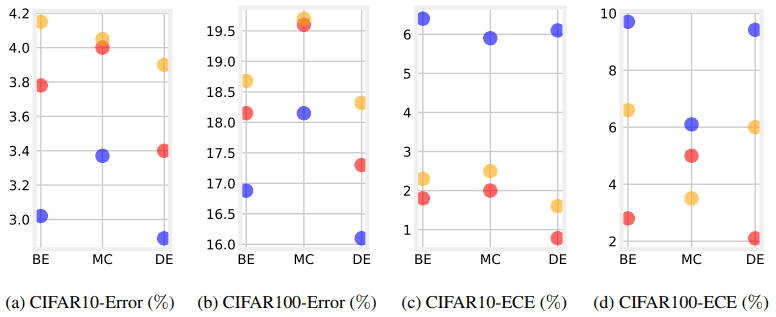These results show the average of the results run for the five random seeds. Red is for ensemble only, blue is for Mixup + ensemble, and orange is for neither. In figures (a) and (b), we can see that the combination of Mixup and Ensemble improves the test performance (decreases Error).

On the other hand, Figs. (c) and (d) show that the calibration is worse (ECE is increased) when Mixup is combined with Ensemble.

### Why do Mixup ensembles worsen calibration?

We investigate in more detail the phenomenon of calibration degradation when ensembles are combined with data augmentation. The following figure shows the difference between the average precision and the average confidence calculated for different confidence intervals when BatchEnsemble and Mixup are combined.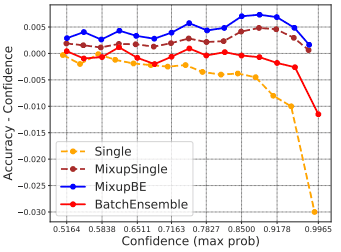If the value of the difference between accuracy and confidence (vertical axis) is positive, it means that the confidence level is low for accuracy (confidence is underestimated), and if it is negative, it means that the confidence level is high (confidence is overestimated).

This figure shows that the accuracy-reliability difference becomes larger and approaches zero for the BatchEnsemble-only and Mixup-only cases compared to the Single network case.

In the case of Misup+BatchEnsemble, the overall accuracy-reliability difference is biased in the positive direction, indicating that the reliability is underestimated relative to the accuracy. In other words, although the data augmentation and ensemble methods have the effect of preventing the overestimation of the confidence level, the simultaneous use of both methods rather underestimates the confidence level, which seems to be the cause of the calibration deterioration.

As a further visualization example, the confidence (softmax probability) of training a three-layer MLP on a simple dataset consisting of five clusters is as follows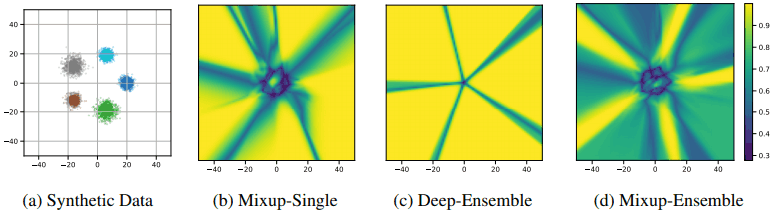In the Mixup/no ensemble case (c), the overall probability is predicted to be high (yellow). This is mitigated by the introduction of Mixup, and by using the ensemble at the same time, we can see that the overall confidence is predicted to be much lower (green).

In addition, label smoothing is one of the most effective methods to suppress overestimation of the confidence level, and the same phenomenon occurs when this label smoothing is used together with an ensemble. The same phenomenon occurs when label smoothing is used together with the ensemble. This is illustrated in the following figure, where the stronger the label smoothing is applied, the larger the increase in ECE.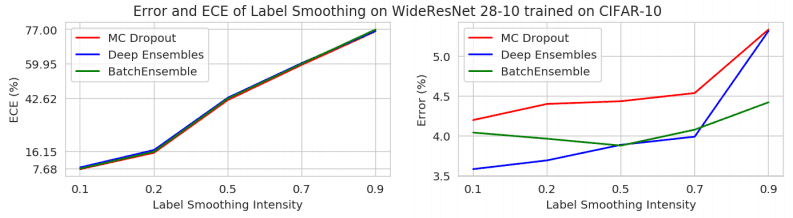## Confidence Adjusted Mixup Ensembles (CAMIXUP)

In the paper, we propose CAMixup as a method to prevent such calibration degradation due to the underestimation of the confidence level. the idea underlying CAMixup is that in a classification task, the difficulty of prediction can vary from class to class. In this case, it is desirable to increase the confidence level in classes where prediction is easy and to prevent the confidence level from increasing too much in classes where prediction is difficult.

CAMixup is based on this idea, and varies the degree of Mixup application for each class, especially for classes where model confidence is likely to be overestimated (difficult to predict). This is illustrated in the following figure.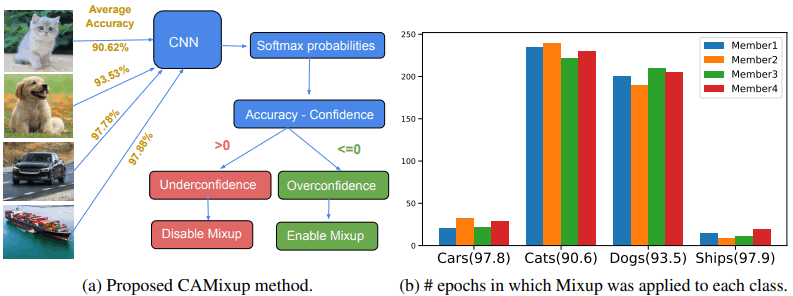As shown in the left figure, if the Accuracy-Confidence difference is positive, Mixup is not applied, and if it is negative, Mixup is applied. In the right figure, the number of times Mixup is applied for each class in 250 epochs is shown. In this case, we can see that Mixup is applied very often to the classes which are difficult to predict (dog and cat). The results of using CAMixup are as follows.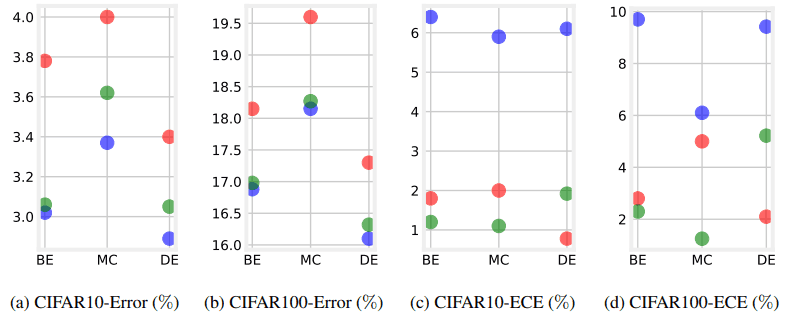Red shows the results for ensemble only, blue for Mixup+ensemble, and green for CAMixup+ensemble. Figures (a) and (b) show that the test accuracy is slightly reduced compared to the regular Mixup, but the ECE can be reduced significantly. In the following table, we also show the results on ImageNet.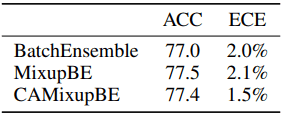It was shown that ECE can be significantly improved while the loss of accuracy is negligible.

Performance during distribution shift

The results of the evaluation with CIFAR-10-C/CIFAR-100-C (C indicates corruption) are as follows.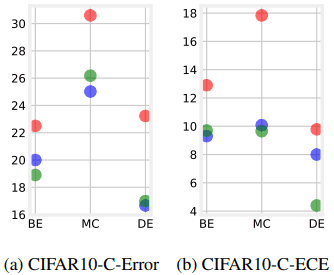As shown in the figure, CAMixup is shown to be effective even in task settings where distributional shifts occur. CAMixup is also shown to work well on AugMix, a state-of-the-art data augmentation method. The results are as follows.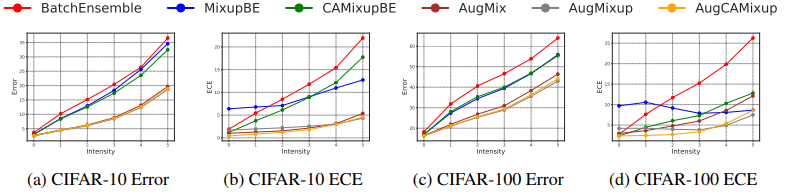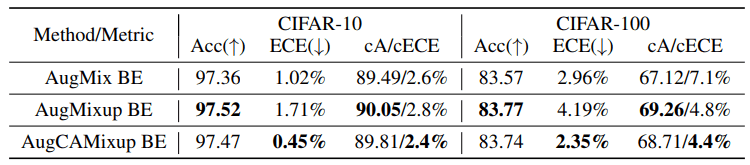AugMixup is a method that combines AugMix and Mixup (details are omitted). The modified version of AugMix combined with CAMixup (AugCAMisup) was shown to improve ECE significantly, as well as the normal CAMixup case.

## Summary

In the paper below, it was shown that calibration can be degraded when ensembles are combined with data augmentation. This is likely due to the fact that ensembles and data augmentation underestimate the confidence level. To avoid this, we proposed CAMixup, which varies the application of Mixup depending on the difficulty of predicting the class.

While both data augmentation and ensembling are effective methods for improving performance, this is an important study in which we discovered a phenomenon that can be harmful by combining them, and showed a solution to it.

If you have any suggestions for improvement of the content of the article,